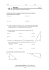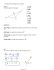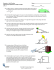# Problem Solving

## Transcription

Problem Solving
```Name ________________________________________ Date __________________ Class__________________
LESSON
8-3
Problem Solving
Solving Right Triangles
that the road rises 28.4 ft over a
horizontal distance of 100 ft. What angle
does the hill make with a horizontal line?
Round to the nearest degree.
_________________________________________
into vehicles usually have slopes
2
1
between
and . What is the range of
5
2
angle measures that most pet ramps
make with a horizontal line? Round to
the nearest degree.
______________________________________
Use the side view of a water slide for Exercises 3 and 4.
The ladder, represented by AB , is 17 feet long.
3. What is the measure of angle A, the
angle that the ladder makes with a
horizontal line?
_______________________________________
4. What is BC, the length of the slide?
Round to the nearest tenth of a foot.
_______________________________________
6. The coordinates of the vertices of RST
are R(3, 3), S(8, 3), and T(8, −6). What is
the measures of angle T? Round to the
nearest degree.
What is the angle that the treadmill
surface makes with a horizontal line?
Round to the nearest degree.
A 3°
C 12°
B 4°
D 31°
C ∠3
B ∠2
D ∠4
H 61°
G 29°
J 65°
8. Find the measure of the acute angle
3
formed by the graph of y = x and the
4
x-axis. Round to the nearest degree.
7. If cos A = 0.28, which angle in the
triangles below is ∠A?
A ∠1
F 18°
F 37°
H 49°
G 41°
J 53°
Original content Copyright © by Holt McDougal. Additions and changes to the original content are the responsibility of the instructor.
8-25
Holt Geometry
United States means a rise in elevation of
20 feet over 100 horizontal feet. A 20%
grade elsewhere means a rise in
elevation of 20 meters over 100 meters.
20 ft
20 m
But a grade is a ratio:
=
.
100 ft 100 m
The units cancel out, and either way a
1
20% grade simplifies to , or an angle
5
with the horizontal that measures about
11°.
6. cos R =
2
; tan R = 1; m∠R = 45°
2
7. sin K =
3
1
; cos K = ; m∠K = 60°
2
2
Problem Solving
6. EG = 0.61 m; FG = 0.54 m; m∠E = 57°
1. 16°
2. 22° to 27°
3. 64°
4. 34.9 ft
5. A
6. G
7. D
8. F
7. KM = 56.13 mm; m∠K = 61°; m∠L = 34°
10. IJ = 5.32 yd; m∠H = 90°; m∠I = 62°
1. finding the measures of all unknown
sides and angles of the triangle
9
2. m∠B = tan−1 4
11. RS = 18.98 mm; ST = 20.07 mm; m∠R =
60°
3. sin 24° =
8. BC = 3.74 ft; m∠B = 83°; m∠D = 48°
9. TV = 8.43 in.; UV = 14.08 in.; m∠T = 79°
Reteach
1. ∠2
2. ∠2
3. ∠1
4. ∠2
5. 53°
6. 79°
7. 74°
8. 12°
4
AB
LESSON 8-4
Practice A
1. horizontal; above
2. depression; below
9. AB ≈ 12.52 ft; AC ≈ 7.54 ft; m∠B = 37°
3. angle of depression
10. FH ≈ 9.12 mi; m∠F ≈ 26°; m∠H ≈ 64°
4. angle of elevation
11. QR ≈ 20.76 km; QS ≈ 25.04 km; m∠Q =
34°
5. angle of depression
12. WX ≈ 18.30 cm; m∠X ≈ 59°; m∠Y ≈ 31°
6. angle of elevation
13. MP = MN = 6; PN ≈ 8.49; m∠M = 90°; m∠P =
45°; m∠N = 45°
9. 11.9 meters
7. 28 feet
14. KL = 7; LJ = 3; JK ≈ 7.62; m∠L = 90°; m∠J ≈
67°; m∠K ≈ 23°
10. 1.9 meters
1. angle of elevation
2. angle of depression
4
2.
3
3. angle of depression
4. angle of elevation
3. 53.1°
5
2 5
4. sin E =
; cos E =
; m∠E ≈ 26.6°
5
5
5. sin M =
8. 35 feet
Practice B
Challenge
3
1.
5
4. 67°; 23°; 12
5. 34 ft 2 in.
6. 37 ft 1 in.
7. 31 ft 10 in.
8. 1.8 m
9. 65°
3
1
; m∠M = 30°
; tan M =
3
2
10. Mr. Shea lives above Lindsey.
Original content Copyright © by Holt McDougal. Additions and changes to the original content are the responsibility of the instructor.
A12
Holt Geometry
```

### installation of an angle board * * * thermopian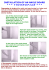### Vector Components Worksheet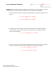### Worksheet - Multi-Step Trigonometry Problem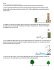### Review12.1-12.6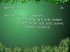### File - HHS Pre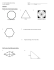### Test 2 Review 1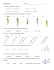### Torque/Cross Product Worksheet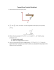### Name______________________________ Worksheet – Solving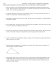### Practice - buskemath